# Counting the Number of Values between Two Specified Values in a List in Microsoft Excel

In this article we will learn how to count the number of values between two specified values in a list in Microsoft Excel.

When you work with reports and dashboards, you are often required to count values b/w a given range. You can use filters to count, but that's not feasible while working with large amounts of data. I mean you don’t want to apply filters on each column again and again to update your report. Right?

Don’t worry, COUNTIFS function will rescue you from all the hassle.

## Static Formula to Count Number of Values Between Two Numbers In Excel 2016

COUNTIFS SYNTAX:

COUNTIFS(Criteria Range1, Criteria, Criteria Range2, Criteria,.....)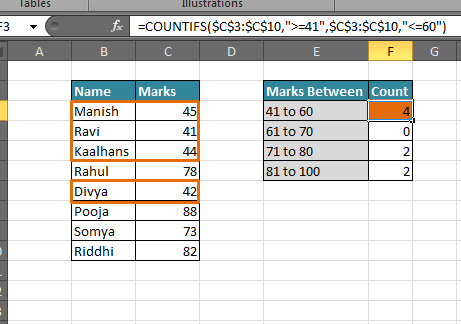Scenario:

Let's say, in a sheet you have a list of students in one column and their marks adjacent to that column. Now you want to lookup all values in excel and count the number of students who scored between: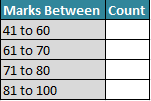Generic Formula:

 =COUNTIFS( SCORE RANGE,”>=START SCORE”, SCORE RANGE, “<=END SCORE” )

The COUNTIFS function of Excel 2016 is that it can handle multiple conditions. In our case, we have only two conditions, the Start Score and End Score.

Implementation:

You want to count the number of students who obtained marks between 41 and 60. Right?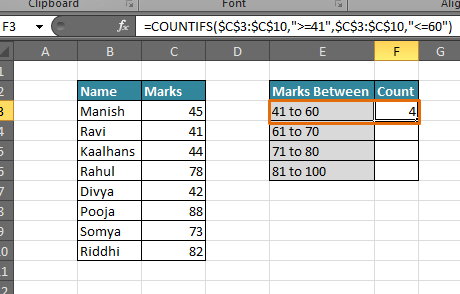To do that we need to Identify all the attributes.

SCORE RANGE: It is the column range that contains marks. That is C3:C10.

START SCORE: The value from which you want to start your account. Or say the lower bound of your range. That is 41.

END SCORE: The value to which you want to count. In other words, the upper bound of your range. That is 60.

In Cell F3, write this formula:

 =COUNTIFS(\$C\$3:\$C\$10,”>=41”, \$C\$3:\$C\$10,”<=60”)

Hit the enter key. You will have your answer. In this scenario, it is 4. There are four students who scored between 41 and 60.

### Explanation:

COUNTIFS can take multiple conditions. It can take more conditions than anyone will ever need, but to be precise, it can handle 127 pairs of ranges and conditions.

Here we have only two conditions, the lower bound and upper bound.

\$C\$3:\$C\$10: So marks are in C3:C10, it means it is our criteria range. We have used \$ before to lock the references. So that it doesn’t change when we copy our formula.

”>=41”: The Lower bound is 41. We used greater than or equal to (>=) sign before 41 so that it includes 41 in our count. Note that we used double quarts for criteria here. This is because we entered the criteria manually.

\$C\$3:\$C\$10: The second criteria range is the same because we want to count from the same column.

”<=60”: The Upper bound is 60. Since we want to include 60 in our result, we used less than or equal to (<=) sign. Now excel will check if a value exists in the data in given range it will count it.

Do the same for each range and It's done.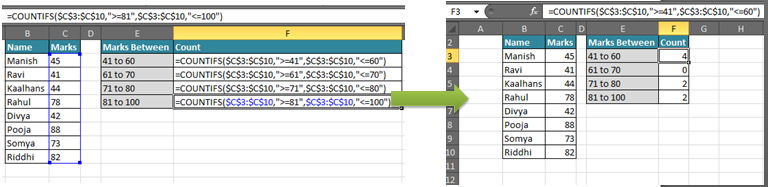In the above example, the formula is hardcoded. If you want add one more range then you need some changes in each formula.

For example, if you enter 100 new conditions then you will need to make 100*2 Changes (Lower bound and Upper Bound). That is why we try not to hard code it.

## Dynamic Formula to Count Number of Values Between Two Numbers

Now we want a formula that counts the number of values between two specified values that is dynamic. We don’t want to make any changes to the new criteria.

Generic Formula:

 =COUNTIFS( SCORE RANGE,”>=”&START SCORE CELL, SCORE RANGE, “<=”&END SCORE CELL)

Implementation:

SCORE RANGE: It is the column range that contains the score. Here it is C3:C10

“>=”: The greater than or equal to sign for checking the values who are greater than or equal to Lower bound.

&: This is very important as it concatenates the cell value with your conditions. “>=”&F3 will translate to “>=41”.

Lower Bound Cell: This is the cell address that contains your lower bound.

Upper Bound Cell: This is the cell address that contains your Upper bound.

In Cell H4, write this

 =COUNTIFS(\$C\$3:\$C\$10,”>=”&F3, \$C\$3:\$C\$10,”<=”&G3)

Hit enter and you have your results.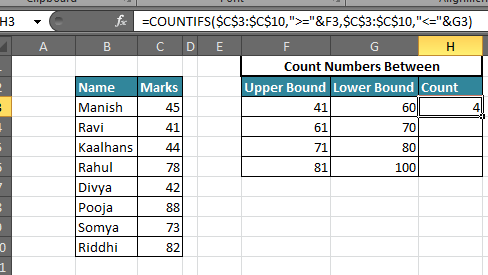Copy the cell H3 in range H4:H6 or just drag it down to H6. You will have all your answers without changing anything in any formula.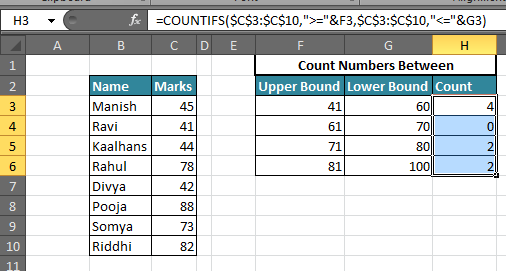Whenever you change to the upper or lower bound, the count will update itself automatically.

Personally, I have used COUNTIFS a lot. For dashboarding, automating reports and many more things. It saves a lot of time. There are many other ways you can use COUNTIFS for doing the same thing.

Related Articles:

How to Count cells which match either A or B in Excel.

How to Count total matches in two ranges in Excel

How to Count cells between values in Excel

How to Count Unique Values In Excel

How to Count Cells That Contain This Or That in Excel

How to do Running Count of Occurance in Excel

Popular Articles:

50 Excel Shortcut to Increase Your Productivity

How to use the VLOOKUP Function in Excel

How to use the COUNTIF function in Excel 2016

How to use the SUMIF Function in Excel

1.???????,??????????! .

s.hina.minsk2.0.15@gmail.com
s.hinam.insk20.1.5@gmail.com
s.hina.mi.nsk2.0.15@gmail.com
sh.inam.i.n.sk2.0.1.5@gmail.com
s.h.in.ami.n.sk2.015@gmail.com

2.???????,??????????! .

p.l.enk.i.s.fi.lm.by@gmail.com

3.Sir.
I have a volume sheet where different types of columns are filled accordingly. However, I need to check the count every hour and update in the same sheet under the given time column (eg: 10:30,11:30,12:30). Eg. I have done 10 items in between 10:30-11:30, I want that to be displayed under 11:30 column, etc. for a 9-hour shift. Kindly help!!!

4.searchmechaniks

Fantastic blog yyou have here but I was wanting to know iff you knew of anyy discussion boards that
to be a part of online community where I can get suggestions from other experienced individuals that share
thee same interest. If you have any recommendations,

5.Sheri Miller

This was very helpful, but I have a similar question. I'm trying to determine the criterium for which at least 90% of my values are beneath. So if I have a list of 20 numbers ranging from 0 to 50, what is the value that 90% of the numbers are below. Is there a way to do that without code?

6.Thank you

7.Use pivot

8.Thanks for the help with countifs and numbers between valus for an excel worksheet. Thought I was going crazy. Your help show me once again how simple excel can be.

Tom Hollister

9.How can i count cells between particular numbers, 1 in this case repeating many times in given range. Example:
1
2
3
4
1
2
1
2
3
4
5
1
2
3

•Hi Ronak,

Let us assume you have the range of numbers in column A starting from A2. Then in B2, write the following function to get the count of number 1.

=COUNTIF(A:A,1)

This will give you the count of number 1 which is 4 as per your above given range.

Terms and Conditions of use

The applications/code on this site are distributed as is and without warranties or liability. In no event shall the owner of the copyrights, or the authors of the applications/code be liable for any loss of profit, any problems or any damage resulting from the use or evaluation of the applications/code.